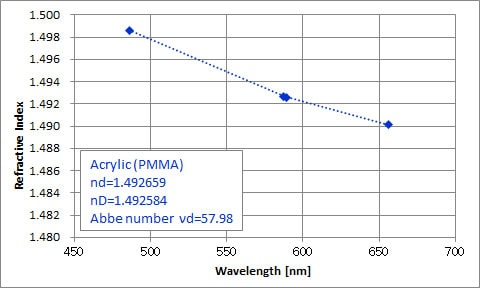Refractive index and dispersion of acrylic resin(PMMA)

Summary

The KPR-300 is the refractometer which can measure the refractive index automatically at multiple wavelengths (d-line, C-line, F-line), and calculate Abbe numbers. The multi-wavelength measurement enables you to acquire the relation between refractive index and wavelength (Δn / Δλ).
The resin material cannot be processed with the accuracy required for spectrometric measurement, so the refractive index cannot be measured with a spectrometer. On the other hand, because of the KPR-300 adopting the V-block method, it can measure the refractive index of the resin with higher precision than an Abbe refractometer.
The refractometer can be used for development of new materials, quality control during the material manufacturing process, and acquisition of the necessary information for optimized design at user intended conditions.

Related information: Measurement of refractive index and dispersion of optical glass (BK7, F2)

Measurement example

 Samples : Acrylic (PMMA) - Molded aspheric lens
 Conditions : Measurement temperature - 23 ° C Measurement wavelength - 3 wavelength (F-line, d-line, C-line) Light source - helium lamp, hydrogen lamp Contact liquid – nd 1.49
 Result : Results of refractive index nd 1.492659 and Abbe number νd 57.98 were obtained. The software of KPR-300 automatically calculates the refractive index nD (589.3 nm, equivalent to sodium D-line) together with the Abbe number when measuring three wavelengths. A refractive index nD of 1.492584 was obtained.Fig1. Refractive index measurement result of acrylic (PMMA/ Molded aspheric lens)

※It is a typical value obtained by this measurement. It does not guarantee the refractive index and the Abbe number of each sample.

No Item Note